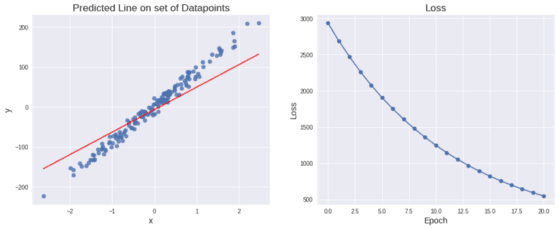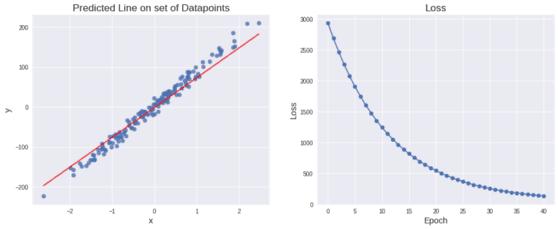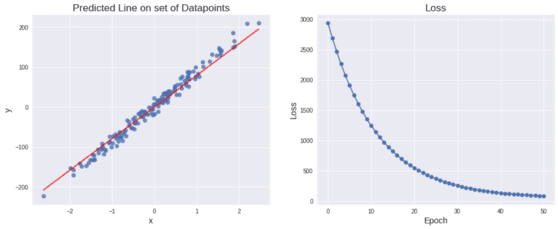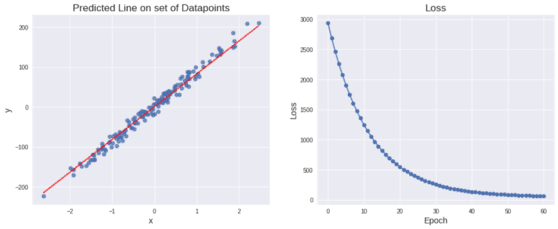# Neural Networks with Numpy for Absolute Beginners — Part 2: Linear Regression

In this tutorial, you will learn to implement Linear Regression for prediction using Numpy in detail and also visualize how the algorithm learns epoch by epoch. In addition to this, you will explore two layer Neural Networks.comments

### Cost/Loss Function

As mentioned earlier, now that you have both the corresponding values for X_train and the predicted values for y_pred you’ll calculate the Cost/Error/Loss Function.

The Loss(Mean Squared Error) is:

Summing over all M examples, we obtain the Loss fn. as below:

Our goal is to obviously minimize the Loss so the regression line predicts more accurately.

Let us now codify this.

You will also save each value of Loss that will be computed to graphically visualize how it changes during training.

def compute_loss(y, y_pred):
loss = 1 / 2 * np.mean((y_pred - y)**2)

return loss

losses = []
loss = compute_loss(y_train, y_pred)
losses.append(loss)
print(loss)

4005.265725705774

Let’s modify the above plot_graph function defined above to plot the Loss too.

def plot_graph(dataset, pred_line=None, losses=None):

plots = 2 if losses!=None else 1

fig = plt.figure(figsize=(8 * plots, 6))

X, y = dataset['X'], dataset['y']

# Plot the original set of datapoints
ax1.scatter(X, y, alpha=0.8)

if(pred_line != None):

x_line, y_line = pred_line['x_line'], pred_line['y_line']

# Plot the randomly generated line
ax1.plot(x_line, y_line, linewidth=2, markersize=12, color='red', alpha=0.8)

ax1.set_title('Predicted Line on set of Datapoints')

else:
ax1.set_title('Plot of Datapoints generated')

ax1.set_xlabel('x')
ax1.set_ylabel('y')

if(losses!=None):
ax2.plot(np.arange(len(losses)), losses, marker='o')

ax2.set_xlabel('Epoch')
ax2.set_ylabel('Loss')
ax2.set_title('Loss')

plt.show()

def plot_pred_line(X, y, m, b,losses=None):

# Generate a set of datapoints on x for creating a line.
# We shall consider the range of X_train for generating the line so that the line superposes the datapoints.
x_line = np.linspace(np.min(X), np.max(X), 10)

# Calculate the corresponding y with the parameter values of m & b
y_line = m * x_line + b

plot_graph(dataset={'X': X, 'y': y}, pred_line={'x_line': x_line, 'y_line':y_line}, losses=losses)

return

You’ll visualize the line created from the parameters m and b.

plot_pred_line(X_train, y_train, m, b,losses)Now that you have computed the loss, let’s minimize it.

### Gradient Descent for Linear Regression

Since, Loss is the dependent variable and m and b are the independent variables, we’ll have to update m & b so as to find the minimum Loss.

So, the immediate question would be…

How can I update the parameters m and b?

Let us for instance consider just a single parameter p as shown below and let t(target) be the value that has to be predicted. We see that as cost converges to the minima, the parameter p reaches a specific value called the optimal value. Let’s say the optimum value of p is a.You can make a few observations from this graph.

It is clear from the graph, that as p moves towards a, the Cost decreases and as it moves away from it, the cost increases.

Now, how can we make p move towards a, regardless of whether it is on the left or to the right of a as shown in figure?

Let us consider the p of the curve. From calculus, we know that the slope of a curve at a point is given by dy/dx (here it is dL/dp where L → Loss). From the fig., when p is to the right of a, the slope is obviously ‒ve and when it’s to the right, the slope would be +ve. But we see that if p is to the left of a, some value must be added to p. Likewise, some value must be subtracted when p is to the right of a.This means that when slope is ‒ve implies p = p + (some val.) and when slope is +ve implies p = p ‒ (some val.) to move towards a.

∴ We subtract the slope from p. This way, the slope is negated and it ensures that it always moves towards a. The resulting equation would be,

It must also be observed that if the cost is too high, the slope will be too high. Hence, while subtracting the slope from p, p value might overshoot a. Hence, it is necessary to decrease the value of slope so that p does not overshoot a. Therefore, we introduce a dampening factor called the Learning Rate (α) to the slope. You’ll see later that by varying α the rate of decrease in error varies.

What we finally obtain would be,

A shown in the figure, the trajectory taken by p against cost is that of a Bell curve.

This method is called the Gradient Descent.

In our case, we use two parameters m and b. Therefore, the Bell curve would be 3-dimensional as shown in the below figure.Gradient Descent w.r.t to parameters m and b.[Source]

As mentioned, you’ll compute the partial derivative of the loss function w.r.t to the parameters m & b. [Note: It is usually expected that you know the basic concepts of partial derivatives. However if you do not, you can refer this wonderful [Khan Academy video]

def gradient(m, b, X_train, y_train, y_pred):

dm = np.mean((y_pred - y_train) * X_train)
db = np.mean(y_pred - y_train)### Updating the parameters

Now we subtract the slope of the parameters m and b from their respective derivatives along with the dampening factor α(alpha).

def update_params(m, b, dm, db, l_r):

m -= l_r * dm
b -= l_r * db

From decreasing the values of m and b, they are incrementally moving towards the minima. So updating the parameters this way has to be done for many iterations, which is called epochs.

Let us define a function grad_desc, which calls both gradient and update_params.

def grad_desc(X_train, y_train, y_pred, m, b, l_r):

dm, db = gradient(m, b, X_train, y_train, y_pred)

m, b = update_params(m, b, dm, db, l_r)

return m, b

We have now defined everything that we need, so let’s compile all the functions into one and see how our algorithm works. So, before you can actually run the code, you’ll have to set the hyperparameters.

# Sample size
M = 200

# No. of input features
n = 1

# Learning Rate - Define during explanation
l_r = 0.05

# Number of iterations for updates - Define during explanation
epochs = 61

X, y = make_regression(n_samples=M, n_features=n, n_informative=n,
n_targets=1, random_state=42, noise=10)

dataset = {'X': X, 'y': y}

plot_graph(dataset)

m, b = init_params()

X, y = reset_sizes(X, y)

X_train, X_test, y_train, y_test = train_test_split(X, y, test_size=0.2)

losses = []

for i in range(epochs):
y_pred = forward_prop(X_train, m, b)

loss = compute_loss(y_train, y_pred)
losses.append(loss)

m, b = grad_desc(X_train, y_train, y_pred, m, b, l_r)

if(i%10==0):
print('Epoch: ', i)
print('Loss = ', loss)Epoch: 0
Loss = 2934.082243250548

Epoch: 10
Loss = 1246.3617292447889Epoch: 20
Loss = 546.310951004311Epoch: 30
Loss = 255.88020867147344Epoch: 40
Loss = 135.36914932067438Epoch: 50
Loss = 85.35744394597806Epoch: 60
Loss = 64.60029693013243Since you have trained the parameters for 60 epochs and the regression line looks to be fitting the data, you can move forward to the last phase, i.e., prediction on our test data and checking the accuracy.

### Prediction

For checking the accuracy, you can take the mean of percentage error for all the test data points.

# Prediction
print('Prediction: ')
y_pred = forward_prop(X_test, m, b)
loss = compute_loss(y_test, y_pred)
print('Loss = ', loss)
accuracy = np.mean(np.fabs((y_pred - y_test) / y_test)) * 100
print('Accuracy = {}%'.format(round(accuracy, 4)))
plot_pred_line(X_test, y_test, m, b)

print('Hence \nm = ', m)
print('b = ', b)


Prediction:
Loss = 56.53060443946197
Accuracy = 80.1676%

Hence
m = 82.34083095217943
b = 0.46491578390750576



The accuracy is 80% which is “ok” considering the variance in the data as is seen in the above graphs.

I was hoping to introduce something really interesting in the article and as a bonus I have also added an intro to Neural Networks. But this surely comes with a catch!

### Two Layer Neural Network with Linear Activation Function

The Neural Network is shown below.From the image, we observe that there are two inputs each to the two neurons in the first layer and an output neuron in the second layer.

We will be using matrices for representing our above equations. We can represent them in vector (single column matrix) form as:

While doing matrix computations, we’ll need to take care of the dimensions and multiply. Hence, we rearrange a bit to arrive at the required output.

The expansion of the equation is not required and hence let’s stick to

Similarly, the value of

Now the output from the 2ⁿᵈ layer will be:

From the above set of equations, we see that a neural network with a linear activation function reduces to a linear equation.

The whole purpose of neural networks was to create a very complex function that can fit to any sort of data and as it can be clearly seen, a neural network with linear activation functions fails the purpose. Hence, it should be strictly noted that a linear function cannot be used as an activation function for the neural network, although it can be used only in the last layer for regression problems.

Then I guess you’ll have to hold your horses until the next tutorial to implement one!

### Here’s the link for the full implementation in Jupyter Notebook:

Go ahead clone it and start running the cells on your Colab to see the miracles of Gradient Descent!!

### Conclusion

In this tutorial, you learnt

1. Linear Activation functions perform the tasks of regression i.e., learn to predict and forecast values. This method is called Linear Regression everywhere.
2. An MLP(Multi-Layer Perceptron) with a linear activation function reduces to a normal Linear Regression task. Hence, linear activations must not be used in the hidden layers of a network. However, it can be used in the last layer for regression/prediction tasks.

In the next tutorial, you’ll learn about Sigmoid Activation Function and perform Logistic Regression which is the most important key to implement neural networks.

Are you working on any cool Deep Learning project?

OR message me on Twitter for any queries: Suraj Donthi (@suraj_donthi) | Twitter

Bio: Suraj Donthi is a Computer Vision Consultant | Author | Machine Learning and Deep Learning Trainer.

Original. Reposted with permission.

Related: